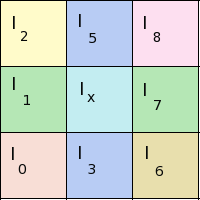## Thursday, June 3, 2010

This time i will write short edge detection tutorial for OpenGL GLSL language. Suppose we have 9 pixels such as below :We want to find out intensity of pixel I_x after applying edge detection filter. In simple edge detection model intensity I_x can be defined as:That is - for being able to calculate pixel intensity we need to find out intensity differences between neighboring pixels and average them. Pixel shader program which implements this idea is given below (In GLSL language):

`uniform sampler2D tex;float threshold(in float thr1, in float thr2 , in float val) { if (val < thr1) {return 0.0;} if (val > thr2) {return 1.0;} return val;}// averaged pixel intensity from 3 color channelsfloat avg_intensity(in vec4 pix) { return (pix.r + pix.g + pix.b)/3.;}vec4 get_pixel(in vec2 coords, in float dx, in float dy) { return texture2D(tex,coords + vec2(dx, dy));}// returns pixel colorfloat IsEdge(in vec2 coords){  float dxtex = 1.0 / 512.0 /*image width*/;  float dytex = 1.0 / 512.0 /*image height*/;  float pix;  int k = -1;  float delta;  // read neighboring pixel intensities  for (int i=-1; i<2; i++) {   for(int j=-1; j<2; j++) {    k++;    pix[k] = avg_intensity(get_pixel(coords,float(i)*dxtex,                                          float(j)*dytex));   }  }  // average color differences around neighboring pixels  delta = (abs(pix-pix)+          abs(pix-pix) +          abs(pix-pix)+          abs(pix-pix)           )/4.;  return threshold(0.25,0.4,clamp(1.8*delta,0.0,1.0));}void main(){  vec4 color = vec4(0.0,0.0,0.0,1.0);  color.g = IsEdge(gl_TexCoord.xy);  gl_FragColor = color;}`

So by using this shader this image =>is transformed into this =>For more advanced algorithms on edge detection - start here.

Have fun with pixel shaders !

1.Thanks for this simple but effective edge detection!

2.I know this was 8 years ago, but do you happen to have an updated solution for this? In the latest WebGL, index expression must be constant, so this code produces an error.

3.Sorry no, I don't work in shader/image processing field anymore

Comment will be posted after comment moderation.